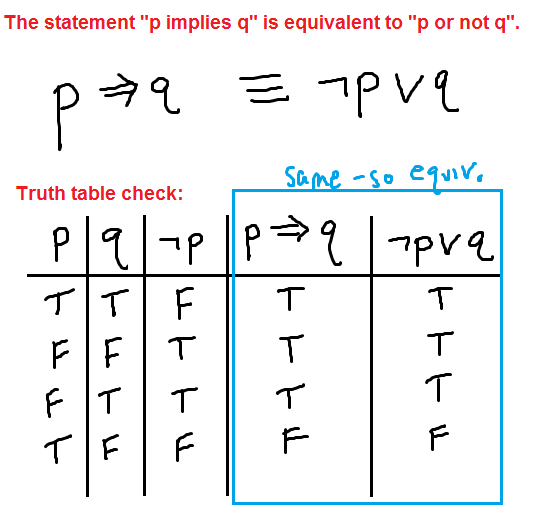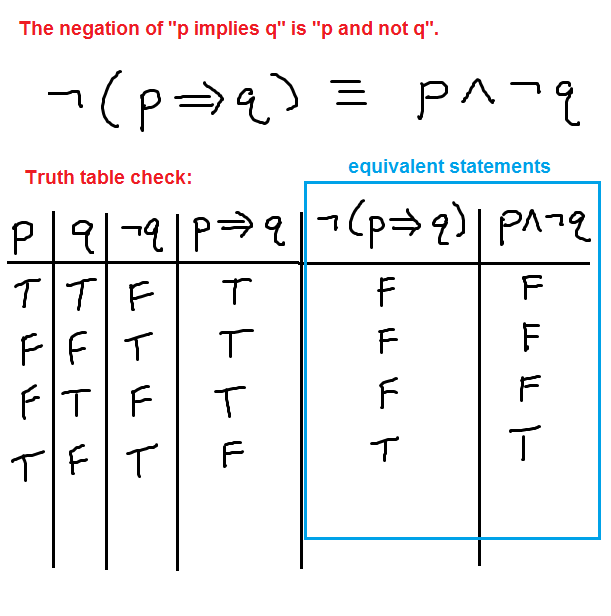# Negating the conditional if-then statement p implies q

The negation of the conditional statement “p implies q” can be a little confusing to think about. But, if we use an equivalent logical statement, some rules like De Morgan’s laws, and a truth table to double-check everything, then it isn’t quite so difficult to figure out. Let’s get started with an important equivalent statement to the conditional.

One way to write the conditional is: “if p, then q”. Thus, if you know p, then the logical conclusion is q. Consider this as you review the following truth table.Why is this true? Given “p implies q”, there are two possibilities. We could have “p”, and therefore “q” (so q is possibility 1). Or, we could have “not p”, and therefore, we would not have q (so we could use possibility 2 as not p). Thus, “p implies q” is equivalent to “q or not p”, which is typically written as “not p or q”. This is one of those things you might have to think about a bit for it to make sense, but even with that, the truth table shows that the two statements are equivalent.

## Using this to negate the statement

Now we can use De Morgan’s laws to negate this statement:

\begin{align} \neg \left(p \implies q\right) &\equiv \neg \left(\neg p \lor q \right)\\ &\equiv \neg \neg p \land \neg q\\ &\equiv p \land \neg q\end{align}

This shows that the negation of “p implies q” is “p and not q”. If we were to apply this to a real-life statement, then we would have something like the following.

Statement: If I run fast, then I get tired. (p implies q)

Negation: I run fast and I do not get tired. (p and not q)

## Verifying with a truth table

Although the work above is enough, you can always double check your results using a truth table. Let’s try it for this negation.As you can see, we end up with the same truth values for each statement, so they are equivalent and we have verified that we did the negation correctly.